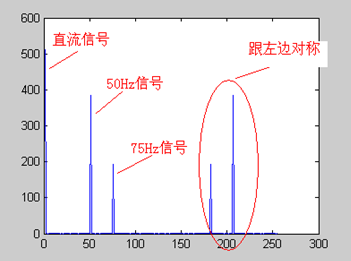FFT是离散傅立叶变换的快速算法，可以将一个信号变换到频域。有些信号在时域上是很难看出什么特征的，但是如果变换到频域之后，就很容易看出特征了。这就是很多信号分析采用FFT变换的原因。另外，FFT可以将一个信号的频谱提取出来，这在频谱分析方面也是经常用的。

${F_n} = \frac{ {(n - 1) \times {F_s}} }{N}$

${A_n}=\sqrt{ {a^2}+{b^2} }$

$\frac{ { {A_n} } }{ {N/2} } \times \cos (2\pi \times {F_n} \times t + {P_n})$

$S=2+3*cos(2*\pi*50*t-\pi*30/180)+1.5*cos(2*\pi*75*t+\pi*90/180)$1点： 512+0i 2点： -2.6195E-14 - 1.4162E-13i 3点： -2.8586E-14 - 1.1898E-13i

50点：-6.2076E-13 - 2.1713E-12i 51点：332.55 - 192i 52点：-1.6707E-12 - 1.5241E-12i

75点：-2.2199E-13 -1.0076E-12i 76点：3.4315E-12 + 192i 77点：-3.0263E-14 +7.5609E-13i

1点： 512 51点：384 76点：192

close all; %先关闭所有图片
A1=3;   %频率F1信号的幅度
A2=1.5; %频率F2信号的幅度
F1=50;  %信号1频率(Hz)
F2=75;  %信号2频率(Hz)
Fs=256; %采样频率(Hz)
P1=-30; %信号1相位(度)
P2=90;  %信号相位(度)
N=256;  %采样点数
t=[0:1/Fs:N/Fs]; %采样时刻

%信号

%显示原始信号
plot(S);
title('原始信号');

figure;
Y = fft(S,N); %做FFT变换
Ayy = (abs(Y)); %取模
plot(Ayy(1:N)); %显示原始的FFT模值结果
title('FFT 模值');

figure;
Ayy=Ayy/(N/2);   %换算成实际的幅度
Ayy(1)=Ayy(1)/2;
F=([1:N]-1)*Fs/N; %换算成实际的频率值
plot(F(1:N/2),Ayy(1:N/2));   %显示换算后的FFT模值结果
title('幅度-频率曲线图');

figure;
Pyy=[1:N/2];
for i="1:N/2"
Pyy(i)=phase(Y(i)); %计算相位
Pyy(i)=Pyy(i)*180/pi; %换算为角度
end;

plot(F(1:N/2),Pyy(1:N/2));   %显示相位图
title('相位-频率曲线图');